# V4F2 Markov Processes

### WS 2017/2018

Tuesday 16.15-17.45 and Thursday 10.15-11.45, Zeichensaal, Wegelerstr. 10.

Tutorial classes: Nikolay Barashkov, Robert Crowell / Group 1: Mon 12-14 N0.007(Neubau) and Group 2: Wednesday 8-10 SemR 1.008.

Exam: 13/2-15/2 and 4/4-6/4

Topics

• Basic theory of Markov processes, strong Markov property.
• Markov chains in discrete time (Generator, martingales, recurrence and transience, Harris Theorem, ergodic averages, central limit theorem)

### Prerequisites

Basic measure theory, conditional expectations, discrete time martingales, Brownian motion.

The lecture notes of Prof. Bovier SS2017 foundations course on Stochastic Processes are available here (pdf). There you find all the necessary background material.

### Lecture Notes

The first part of the course will be mainly based on Prof. Eberle's lecture notes for Markov processes WS16/17 (pdf). Some notes for the lectures will be posted here:

• Note 1 : Introduction, examples, the canonical setup and the strong Markov property. (pdf) [version 1.1, posted 24/10/2017]
• Note 2 : Martingale connection, recurrence of discrete Markov chains, Forster-Lyapounov criteria for recurrence. [version 1, posted 2/11/2017]

### Further References

• Ligget: Continuous Time Markov Processes, AMS
• Chung: Lectures from Markov Processes to Brownian Motion, Springer
• Eberle: Lecture notes for the course "Markov Processes"

### Course Journal

• Lecture 10/10 : Overview of the course. Definition of a Markov process. Transition kernels.
• Lecture 12/10 : Contruction of a Markov process via Kolmogorov's theorem. General Markov property via the shift operator. Examples.
• Lecture 24/10 : Other examples of Markov processes: Brownian motion and the Poisson process. The need for the canonical setup. Feller property and the right continuous filtration.
• Lecture 26/10 : Stopping times. The Strong Markov property. Examples where the strong Markov property does not hold.
• Lecture 2/11 : Zeros of the Brownian motion. Martingale problems for Markov chains.
• Lecture 7/11 : Lyapounov functions, recurrence properties via supermartingales of the Markov chain.
• Lecture 9/11 : Recurrence and transience of discrete Markov chains. Classification of states and irreducibility. Forster-Lyapounov criteria for recurrence.
• Lecture 14/11 : Recurrence for chains in general state spaces. Weak convergence in Polish spaces. Existence of invariant measures.
• Lecture 16/11 : Compactness and weak convergence. Tightness, Prohorov theorem. Krylov-Bogoliubov theorem.
• Lecture 21/11 : Lyapounouv function criteria for existence of invariant measures. Martingale problem in continuous time. Explosion and stability.
• Lecture 23/11 :  Potential theory and Lyapounov functions for  stability and invariant measures in continuous time. Euler approximation. (Eberle, Section 1.4)
• Lecture 28/11 : Ergodic theorems for Markov processes. Shift invariant sigma algebra. Ergodicity and some examples.
• Lecture 30/11 : Proof of Birkhoff ergodic theorem.
• Lecture 5/12 : Ergodic theorems for Markov chains.
• Lecture 7/12 : Central limit theorems for ergodic averages of Markov chains.
• Lecture 12/12 : Structure of invariant measures. Couplings and Wasserstein distance.
• Lecture 14/12 : Optimal couplings, proof of the distance property, Kantorovich duality
• Lecture 19/12 : Contraction coefficients, Path coupling method. Markov chain monte Carlo, detailed balance condition, Metropolis and Glauber dynamics.
• Lecture 21/12 : Geometric ergodicity of Gibbs sampler. Quantitative bounds for ergodic averages. Total variation norm.
• Lecture 9/1 : TV contraction coefficients. Harris' theorem.
• Lecture 11/1 : Coupling of Markov processes. Convergence rates via coupling. CLT for non stationary Markov processes via coupling.
• Lecture 16/1 : Construction of Markov processes with bounded jump rates.
• Lecture 18/1 : Proof of the Markov property and identification of the generator.
• Lecture 23/1 : Some Markov processes with unbounded jump rates: particle systems.
• Lecture 25/1 : Proof of the Markov property and identification of the generator. Attractive particle system and their invariant measures.
• Lecture 30/1 :
• Lecture 1/2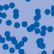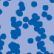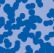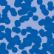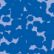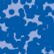## News

Der SFB 1060 Die Mathematik der emergenten Effekte hat eine dritte Förderperiode erhalten. (26.11.20)

Prof. Dr. Andreas Eberle erhält den diesjährigen Lehrpreis der Universität Bonn. (22.07.2020)

Herr Dr. Richard Höfer erhielt den Hausdorff-Gedächtnispreis 2019 der Fachgruppe Mathematik für die beste Disseration. Betreut wurde die Arbeit von Prof. J. Velázquez (29.01.2020).

Einer der Preise der Bonner Mathematischen Gesellschaft (BMG) für die besten Bachelorarbeiten 2019 ging an Herrn Peter Holderrieth unter der Betreuung von Prof. A. Eberle (29.01.2020).

Contact

Managing Director: Prof. Dr. Massimiliano Gubinelli